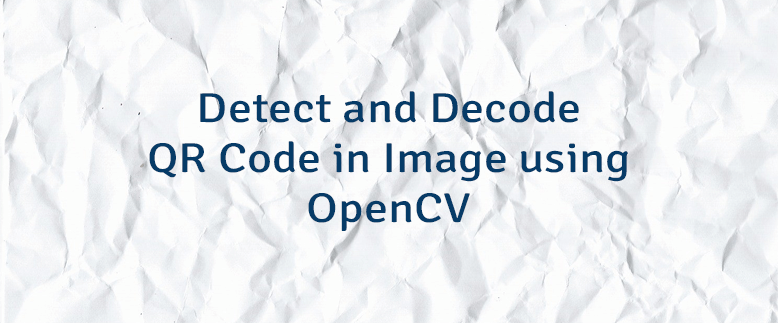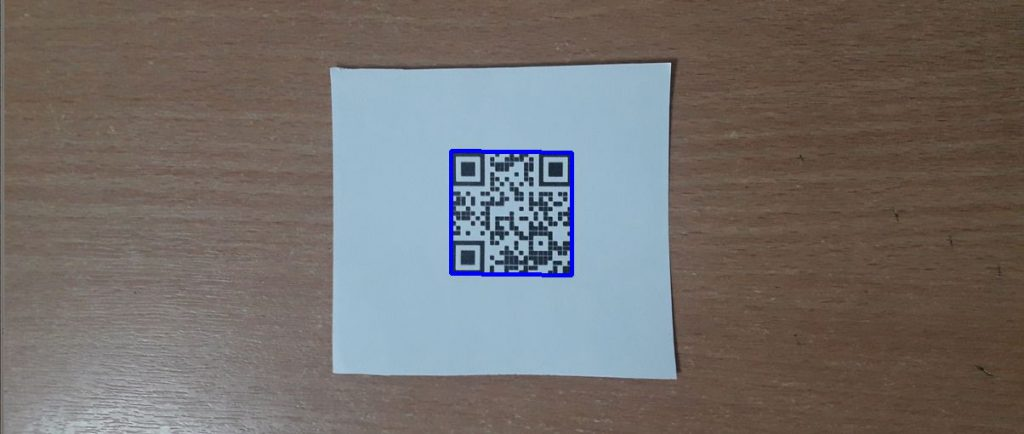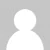# Detect and Decode QR Code in Image using OpenCV

QR code is a two-dimensional barcode which stores encoded data. It can be a website URL, contact details, location coordinates, email address, plain text, etc. QR code can store more data than a linear barcode of equal size.

This tutorial provides an example how to detect and decode a QR code in an image using OpenCV.

We create an object of class `QRCodeDetector`. QR code is detected and decoded by using the `detectAndDecode` method. It allows getting decoded data and an array of vertices of the found QR code.

``````import cv2

decoder = cv2.QRCodeDetector()
data, points, _ = decoder.detectAndDecode(img)

if points is not None:
print('Decoded data: ' + data)

points = points
for i in range(len(points)):
pt1 = [int(val) for val in points[i]]
pt2 = [int(val) for val in points[(i + 1) % 4]]
cv2.line(img, pt1, pt2, color=(255, 0, 0), thickness=3)

cv2.imshow('Detected QR code', img)
cv2.waitKey(0)
cv2.destroyAllWindows()``````
``````#include <iostream>
#include <opencv2/opencv.hpp>

using namespace cv;

int main()
{

QRCodeDetector decoder = QRCodeDetector();
std::vector<Point> points;
std::string data = decoder.detectAndDecode(img, points);

if (!points.empty()) {
std::cout << "Decoded data: " << data << std::endl;

for (int i = 0; i < points.size(); i++) {
Point pt1 = points[i];
Point pt2 = points[(i + 1) % 4];
line(img, pt1, pt2, Scalar(255, 0, 0), 3);
}

imshow("Detected QR code", img);
waitKey(0);
destroyAllWindows();
}

return 0;
}``````
``````package app;

import org.opencv.core.*;
import org.opencv.highgui.HighGui;
import org.opencv.imgcodecs.Imgcodecs;
import org.opencv.imgproc.Imgproc;
import org.opencv.objdetect.QRCodeDetector;

public class Main
{

public static void main(String[] args)
{

QRCodeDetector decoder = new QRCodeDetector();
Mat points = new Mat();
String data = decoder.detectAndDecode(img, points);

if (!points.empty()) {
System.out.println("Decoded data: " + data);

for (int i = 0; i < points.cols(); i++) {
Point pt1 = new Point(points.get(0, i));
Point pt2 = new Point(points.get(0, (i + 1) % 4));
Imgproc.line(img, pt1, pt2, new Scalar(255, 0, 0), 3);
}

HighGui.imshow("Detected QR code", img);
HighGui.waitKey(0);
HighGui.destroyAllWindows();
}

System.exit(0);
}
}``````

If a QR code was found, we print the decoded data and draw a bounding box around the detected QR Code.

``Decoded data: https://lindevs.com``1.Thanks You !! u r code is perfect ..i am using this code

2.